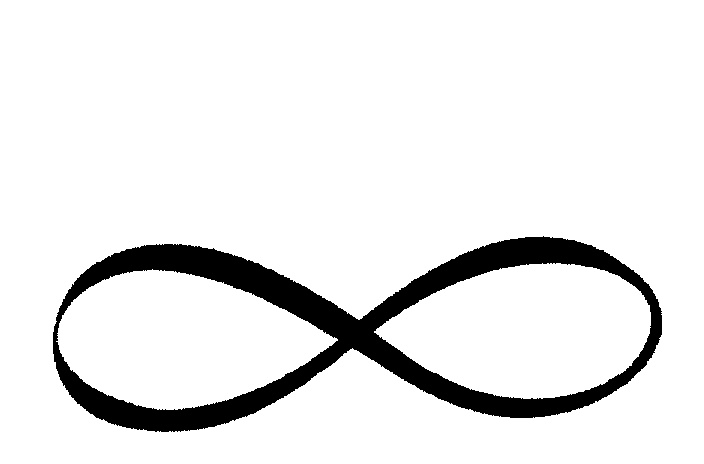Posted on May 16, 2016

# A gentle introduction to infinities

As children, we learn about infinity in much the same way we learn to tie our shoes. First, we are taught an complicated sequence of motions and symbols, with little explanation of why the rabbit has to go around the tree before it jumps into the hole. It is only later after learning an appreciable amount of physics and geometry that the reason one’s shoes do not fall off becomes clear. One spends the majority of grade school mathematics classes shunting symbols about a page, with little appreciation for the meaning of the odd sideways eight.Although there is much to be said about the complexity of shoe-tying, I would argue that the notion of infinity is slightly more difficult to comperhend. As a child, I used the word infinity to mean something “really really really big” when I was too lazy to say the word “really” that many times. Occasionally I simply threw the word about because I thought it made me sound precocious. Of late, however, coursework has required me to give the subject deeper thought.

Computer scientists frequently find themselves dealing with unfathomably large numbers. Many concepts in theoretical computer science – in particular, the analysis of algorithmic complexity – rely on taking limits as the size of the input to a problem becomes ‘infinitely’ large. Yet too often the subject of size is approached in a hand-wavey fashion that leaves a slight distaste in the mouth of a student in mathematics. Computer science students, it seems, often find themselves forging ahead without first checking whether their mathematical shoes are securely fastened. In this post, I’ll attempt to make some small effort at remedying this worrying trend, and try to explain one small facet of the concept of infinity.

I’ll start with FizzBuzz, the classic programming interview question, which goes as follows. Loop through a set of numbers. If a number is divisible by 3, print “Fizz”. If it’s divisible by 5, print “Buzz”. In python, this would look like:

a = [1,2,3]
a = a
print(a)
>>> [1,2,[...]]

Now, one question we might ask about this program is “How many times will it print fizz in a given input?”, and we’d find that the answer is $$\rfloor \frac{n}{3} \lfloor$$ ( $$\rfloor 3.2 \lfloor = 3$$, as an example). Similarly, we’ll print “buzz” $$\rfloor \frac{n}{5} \lfloor$$ times.

Now, imagine we had a computer that could store arbitrarily large numbers and had it run the following, slightly modified version of the fizzbuzz solution:

i = 1
while i >0:
if i%3==0:
print "fizz"
if i % 5 == 0:
print "buzz"
i++;

It doesn’t take much contemplation to recognize that this program won’t terminate any time soon (barring the heat death of the universe). So if the universe doesn’t end, and our program runs forever, will we have printed the word “fizz” more times, or the word “buzz”?

The answer is subtle. If we stop the computer at any point, the answer will be that we printed “fizz” more often, since there are more numbers that are divisible by 3 than by 5 in the interval (1, n) for any value of n. Intuitively, we might then look at this output and say that since the ratio of fizz to buzz favours fizz, there are more whole numbers divisible by 3 than by 5.

As appealing as this explanation is, it doesn’t hold water since our program doesn’t terminate. The number of fizzes is $$(3)( \infty) \leq (5) (\infty)$$ (the number of “buzz”es), but since $$(3 )(\infty) = \infty$$ and $$(5) (\infty) = \infty$$, we’re left with $$\infty < \infty$$, which is just silly.

Instead, I’m going to propose that we use a different way of comparing the two numbers. When we compare two sets of objects, we can either count one set then count the other and compare our final totals for each, or we can pair off elements from the two sets and see what’s left over.

Now the question becomes “how do we pair off elements of infinitely big sets?”. The smallest number in each set (we can use the ever-useful well-ordering property for natural numbers) seems like a good candidate. That would be the pair 3, 5. Then we pair off 6, 10, then 9, 15, and so on. If you see the pattern already, great, otherwise stare at the numbers for a little while until it dawns on you (or read on if you’re feeling lazy).

The pattern for pairs (x,y) can be easily written as the function $$y = \frac{5x}{3}$$. Furthermore, when we use this approach for pairing, each “fizz” gets paired off with a “buzz”, and each “buzz” is paired with a “fizz”. So if we continued to pair them off like so forever, we wouldn’t have any “buzz”es left over.

If you’re not convinced of this fact, consider what would happen if we did have a buzz left over. Then this buzz would be associated with some number n from when we ran the program. Since $$n$$ is a finite multiple of 5, then $$\frac{3n}{5}$$ is also a finite whole number. But this is exactly the number that we were supposed to pair $$n$$ off with! So $$n$$ can’t be left over, and this means that the set of all positive integers that are divisible by 5 is the same size as the set of all positive integers divisible by 3.

This example may seem a bit contrived, but I hope it gave some insight into how infinities work differently from regular numbers.